• 接上文：Matlab精彩画图...这里有种方式：利用plot指令同时绘制多条曲线；利用hold on命令叠加绘制多条曲线。1、plot 命令同时绘制多条曲线Matlab代码：如上图所示，plot指令可同时绘制多条曲线。运行结果：2、h...接上文：Matlab精彩画图示例：多子图Matlab精彩画图示例：图上图对比查看不同曲线，除了采用图上图、多子图的方式，还可以采用将多条曲线画到一张图里查看，这样更方便、直观，尤其对于曲线族的绘制。这里有两种方式：利用plot指令同时绘制多条曲线；利用hold on命令叠加绘制多条曲线。1、plot 命令同时绘制多条曲线Matlab代码：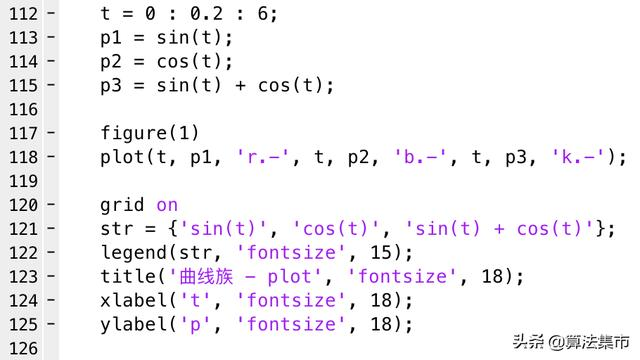如上图所示，plot指令可同时绘制多条曲线。运行结果：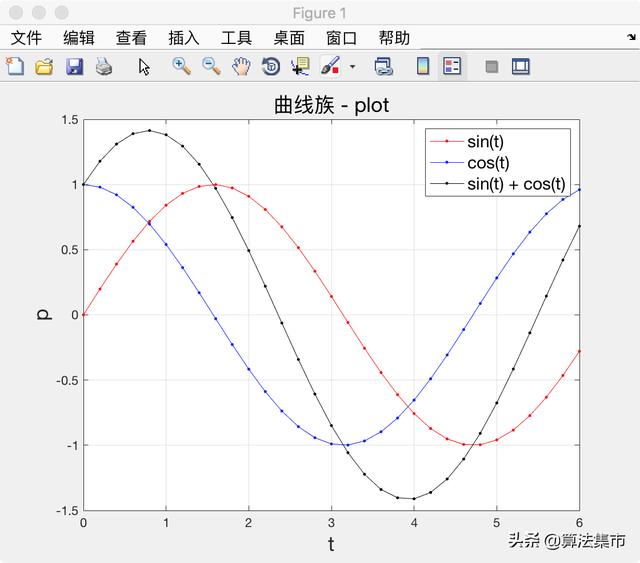2、hold on 命令叠加绘制多条曲线matlab代码：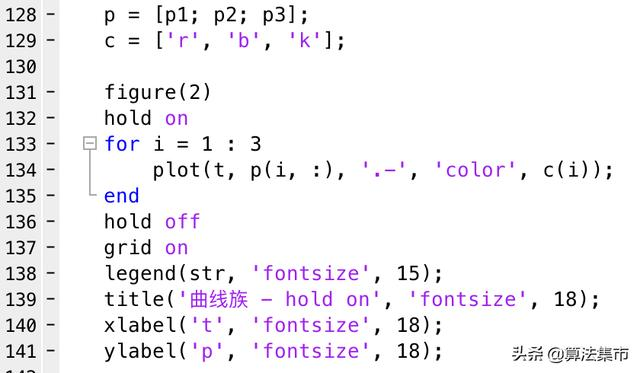如上图所示，可将数据存到一个矩阵里，利用hold on命令可在当前坐标轴上叠加绘制曲线。hold on retains plots in the current axes so that new plots added to the axes do not delete existing plots.程序运行结果：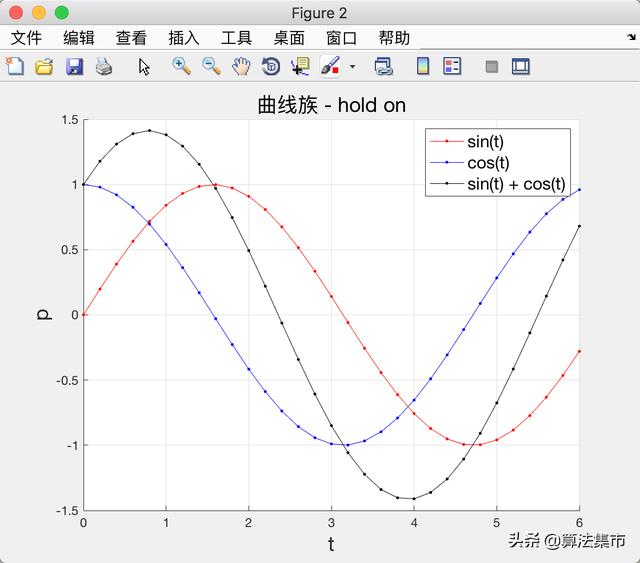需要说明的一点是，即使不设置每条曲线的颜色，matlab也会自动采用不同的颜色来区分不同的曲线。相关阅读Matlab学习总结：基础命令、可视化、算法示例等
展开全文• MATLAB中将曲线画在同一图上，如果直接采用下面的代码，那么画的第二图会将第一图覆盖 plot(x,p1,'LineWidth',2); plot(x,p2,'LineWidth',2); 正确的做法是在条语句中间添加hold on; plot(x,p1,'...
在MATLAB中将两条曲线画在同一个图上，如果直接采用下面的代码，那么画的第二个图会将第一个图覆盖

plot(x,p1,'LineWidth',2);
plot(x,p2,'LineWidth',2);

正确的做法是在两条语句中间添加hold on;

plot(x,p1,'LineWidth',2);
hold on;
plot(x,p2,'LineWidth',2);

展开全文• 本博文源于matlab基础，用例子引入，大家只需要照猫画虎进行套用...例子：绘制曲线y=sin(x+3),y=e^(sin(x))的图形,范围[-2*pi,2*pi] 例子：画出三函数函数y=2*x,y=cos(x),y=sin(x)，范围[-3,3]并作相应的图形标注
本博文源于matlab基础，用例子引入，大家只需要照猫画虎进行套用即可。
绘制两条曲线y=sin(x+3),y=e^(sin(x))的图形,范围[-2pi,2pi]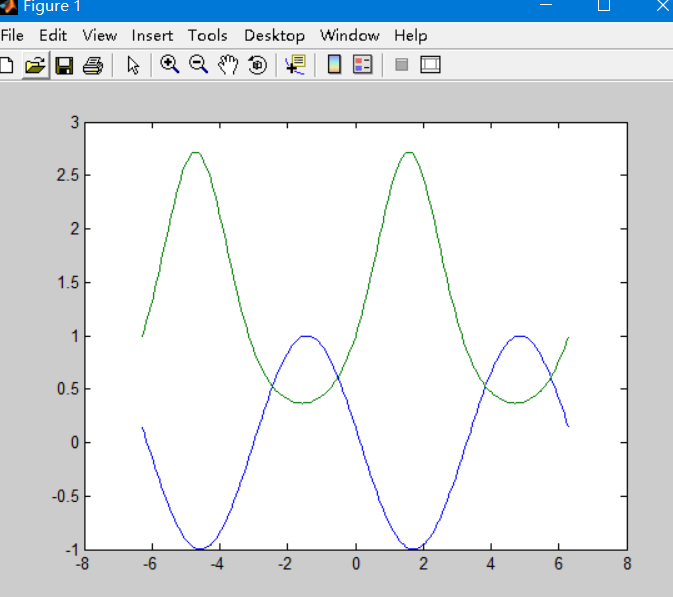这里千万不要直接plot(y)，这样两者作为混合图形，效果既错误又丑陋！
>> x=-2*pi:pi/50:2*pi;
>> y=[sin(x+3);exp(sin(x))];
>> plot(x,y)

画出三个函数函数y=2*x,y=cos(x),y=sin(x)，范围[-3,3]并作相应的图形标注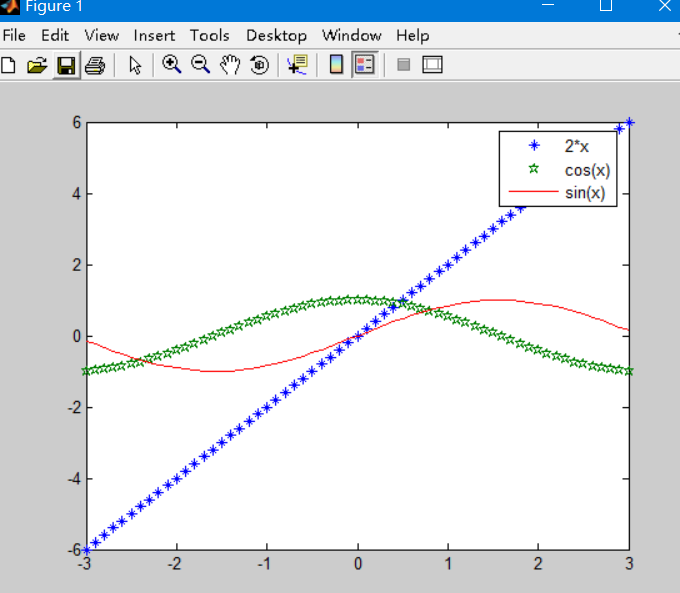>> x=-3:0.1:3;
>> y1=2*x;
>> y2=cos(x);
>> y3=sin(x);
>> plot(x,y1,'*',x,y2,'P',x,y3)
>> legend('2*x','cos(x)','sin(x)')
>>



展开全文数据可视化
• 1.基础绘图和python类似1.1 绘图：plot()如果绘制多条曲线，需要使用hold on-hold off ，不然后面的曲线会覆盖掉前面的...hold off%两个一起画；不使用hold on的话，默认会覆盖掉前面那张图片 1.2 样式plot样式plot(...
1.基础绘图和python类似1.1 绘图：plot()如果绘制多条曲线，需要使用hold on-hold off ，不然后面的曲线会覆盖掉前面的。hold onplot(cos(0:pi/20:2*pi),'o-k');plot(sin(0:pi/20:2*pi),'X--b');hold off%两个一起画；不使用hold on的话，默认会覆盖掉前面那张图片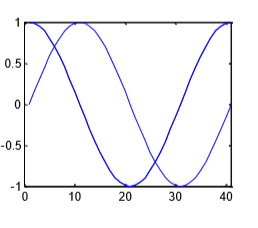1.2 样式plot样式plot(x,y,‘str’)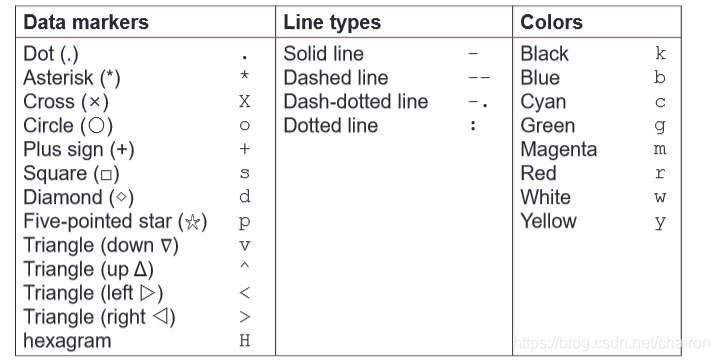图例：legend()title()和xlabel()ylabel()zlabel()x=0:0.5:4*pi;y=sin(x);h=cos(x);w=1./(1+exp(-x));xlabel('t=0 tp 2\pi');ylabel('values of sin(t) and e^{-x}');plot(x,y,'bd-',x,h,'gp:',x,w,'ro-',x,w,'c^-');%样式title('values of sin(t) and e^{-x}');%标题legend('sin(x)','cos(x)','sigmoid','Gauss function');%图例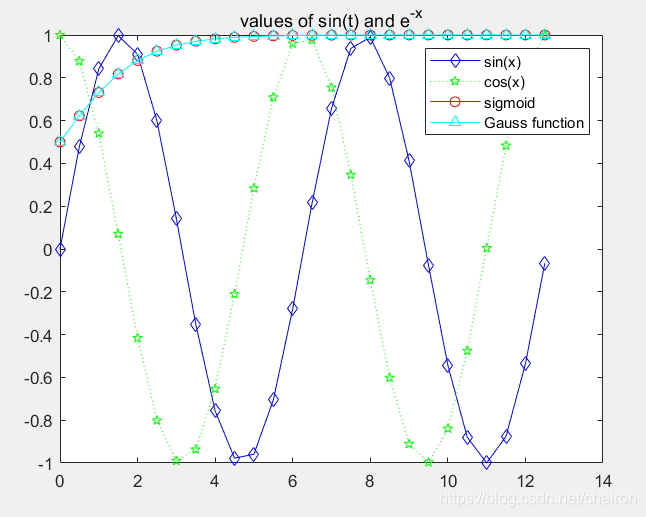text() and annotation()数学公式使用latexx = linspace(0,3);y = x.^2.*sin(x);plot(x,y);line([2,2],[0,2^2*sin(2)]);str = '$$\int_{0}^{2} x^2\sin(x) dx$$';%表示积分函数text(0.25,2.5,str,'Interpreter','latex'); %积分函数的位置annotation('arrow','X',[0.32,0.5],'Y',[0.6,0.4]); %箭头位置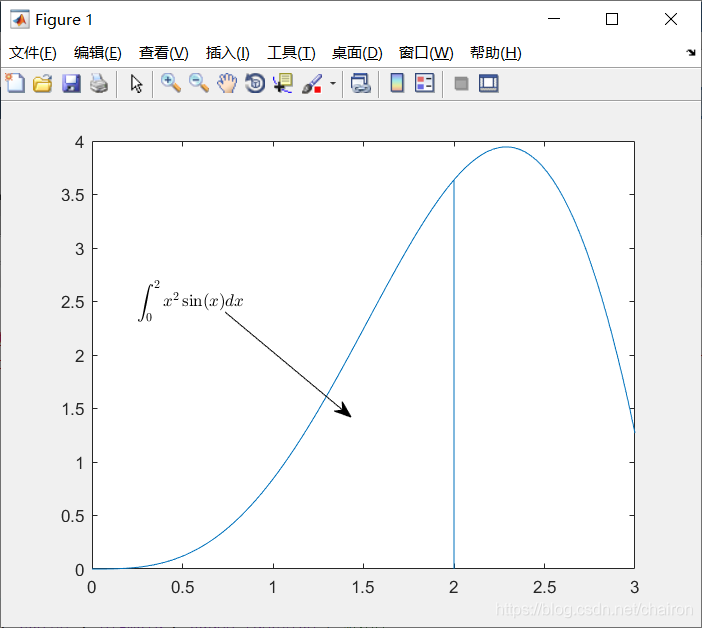小练习：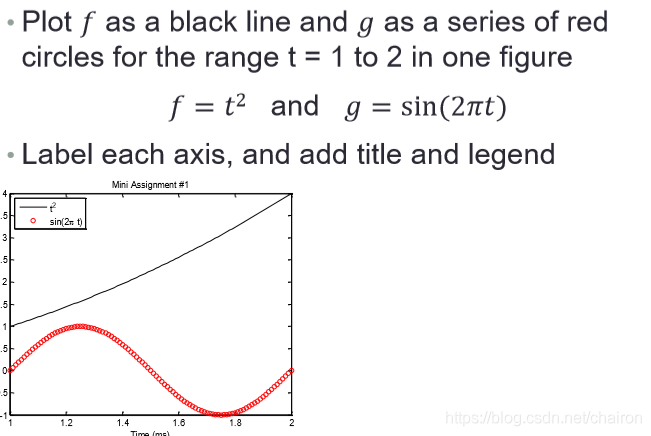t=1:0.01:2;f=t.*t;g=sin(2*pi*t);plot(t,f,'k',t,g,'ro');xlabel('Time (ms)');ylabel('f(t)');title('Mini Assignment #1');legend('t^2','sin(2\pi t)','location','northwest');%northwest指定图例在左上角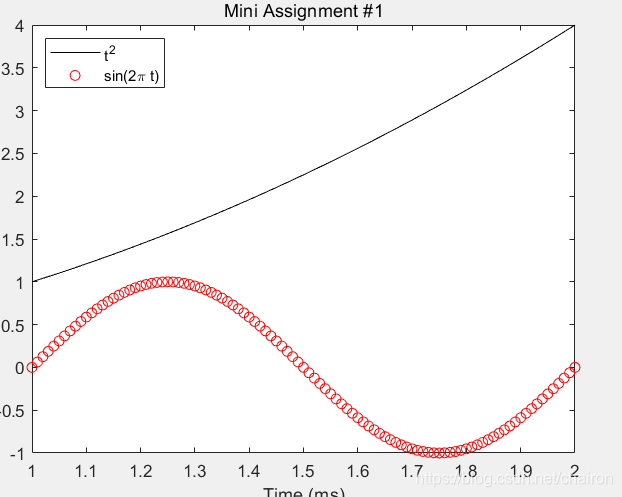2. 图像调整(Figure Adjustment)一张图片由很多对象组成；一个对象又有很多属性。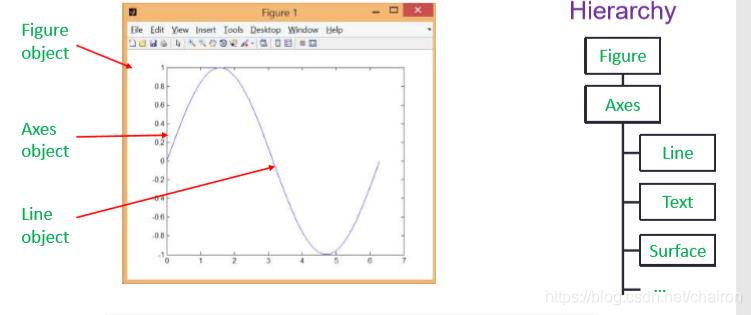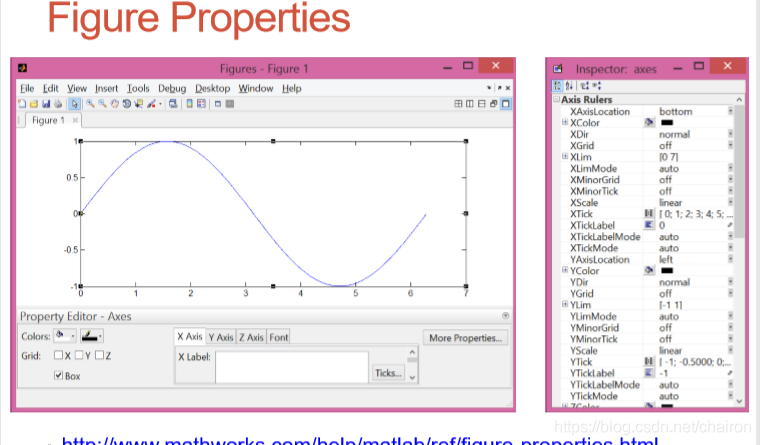2.1 修改图片对象的句柄(handle)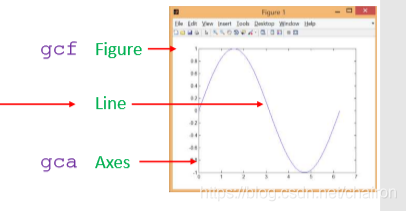函数|函数|解释 ||–|--||gca|返回当前图像的坐标轴axes ||gcf|返回图像的figure对象||allchild|找到具体对象的所有孩子||ancestor|找到图片对象的祖先||delete|删除具体对象||findall|找出所有的图片对象|2.2获得或者修改图片属性获得属性/对象：get()修改属性：set()两者都需要通过获取handle来进行实现。%2020.09.19x = linspace(0, 2*pi, 1000);y = sin(x);h = plot(x,y);get(h) %获取h的信息get(gca)%获取当前图像的axis对象的所有信息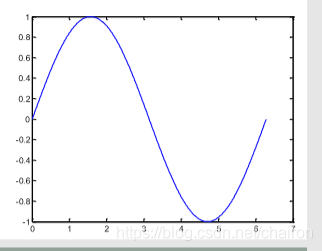%set(gca, 'XLim', [0, 2*pi]);%修改Xlim的取值%set(gca, 'YLim', [-1.2, 1.2]);xlim([0, 2*pi]);%修改Xlim的取值ylim([-1.2, 1.2]);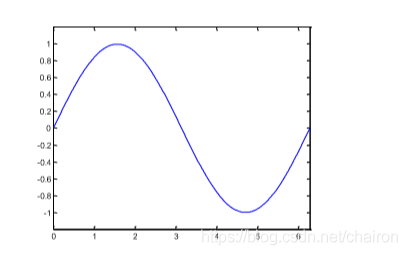2.3 设置字体和坐标轴的间距(tick)set(gca, 'FontSize', 25);%设置字体大小set(gca, 'XTick', 0:pi/2:2*pi);%Tick, YTick, ZTick - 刻度值; 由递增值组成的向量set(gca, 'XTickLabel', 0:90:360);2.4 曲线的格式线宽和曲线样式%set(h, 'LineStyle', '-.',...%    'LineWidth', 7.0, 'Color', 'g');%设置line的格式plot(x,y, '-.g',... 'LineWidth', 7.0)%delete(h);%删掉曲线h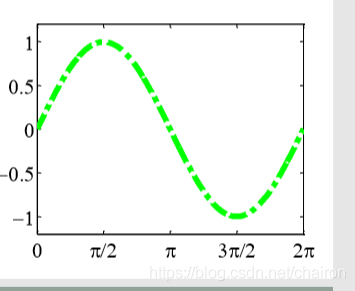图标(maker)的face和edgex=rand(20,1); %rand(3,4) 返回一个 3×4 的矩阵。set(gca, 'FontSize', 18);plot(x,'-md','LineWidth', 2, 'MarkerEdgeColor', 'k',...'MarkerFaceColor', 'g', 'MarkerSize', 10);xlim([1, 20]);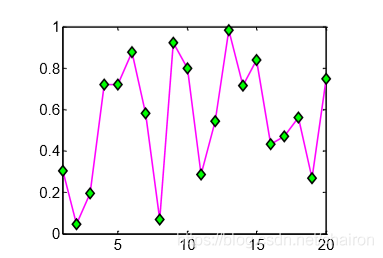2.5 小练习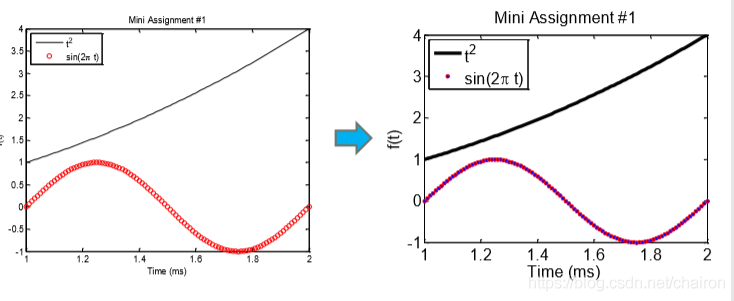t=1:0.01:2;f=t.*t;g=sin(2*pi*t);plot(t,f,'k',t,g,'ro','LineWidth', 2,'MarkerEdgeColor', 'r',...'MarkerFaceColor', 'g');xlabel('Time (ms)');ylabel('f(t)');title('Mini Assignment #1');legend('t^2','sin(2\pi t)','location','northwest');set(gca,'FontSize',20,'FontWeight','bold','TitleFontWeight','bold','XTick', -1:1:4);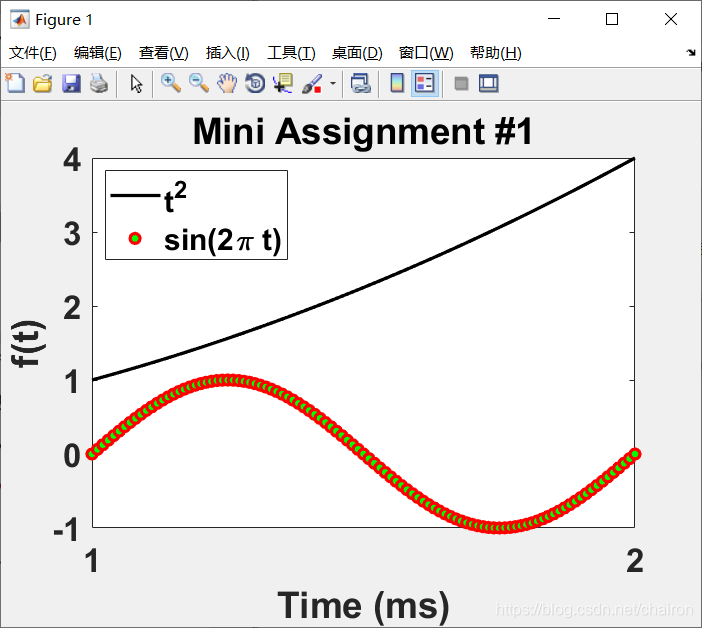3. 组合图片当存在复合图像时，gcf指的是现在的图片，即figure2.x = -10:0.1:10;y1 = x.^2 - 8;y2 = exp(x);figure, plot(x,y1);figure, plot(x,y2);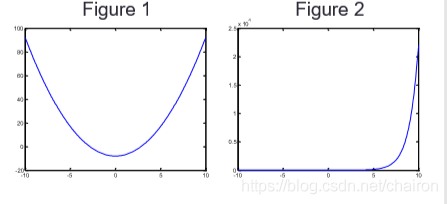3.1 设置图像的位置和大小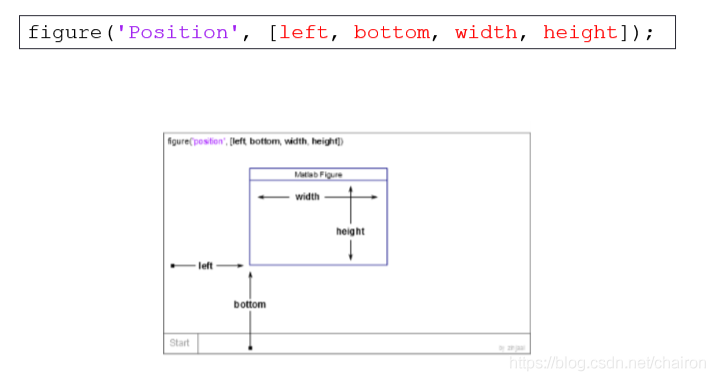3.2 一个figure中显示几个plots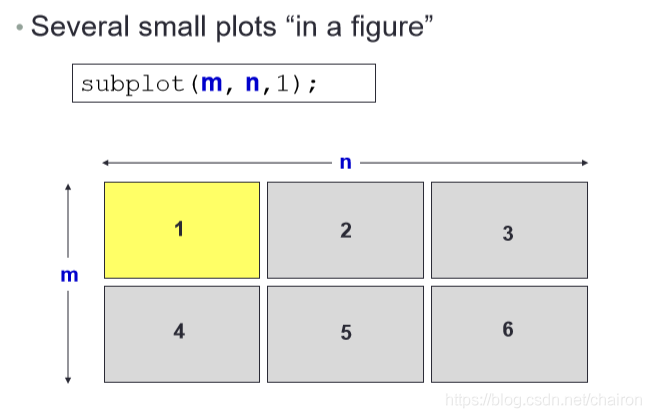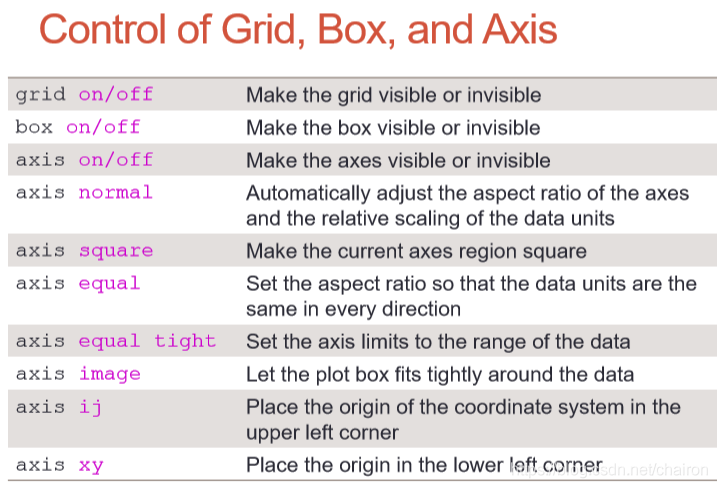t = 0:0.1:2*pi;x = 3*cos(t);y = sin(t);subplot(2, 2, 1);plot(x, y);axis normal %x和y轴自动调整subplot(2, 2, 2);plot(x, y);axis square %x和y轴的总长度一样subplot(2, 2, 3);plot(x, y);axis equal %x和y轴的间隔一样subplot(2, 2, 4);plot(x, y);axis equal tight %Set the axis limits to the range of the dataaxis offaxis on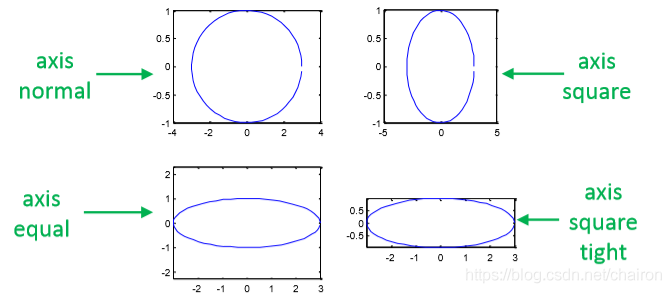4. 把figures保存成文件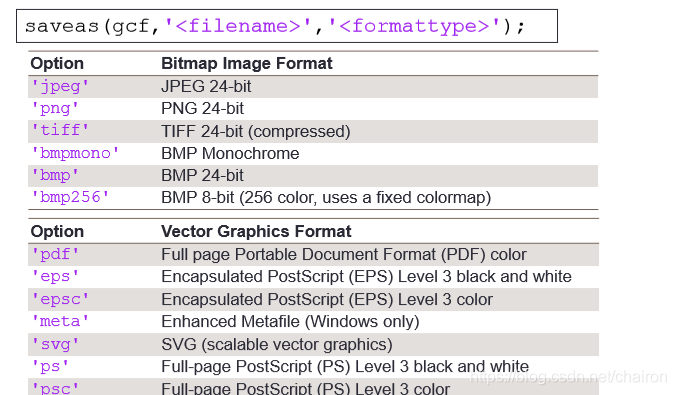如果想要保存成高解析度的文件，建议使用‘print’。%Saving Figures into Filessaveas(gcf,'practice','pdf');原文链接:https://blog.csdn.net/chairon/article/details/108689407
展开全文• MATLAB作图从入门到熟练作为上面这推文的补充。这主要是为了在组会汇报等发表场合，需要用得上动态的标记追寻功能。实现不是很难，主要过程是在我们正常的plot函数后，定义一point，接下来让point的坐标遍历x...
• %多项式曲线拟合 figure(1) matrix2=[]; %新建空矩阵 h1=polyfit(matrix1(:,1),matrix1(:,...plot(matrix1(:,1),matrix2(:,1)) %绘制拟合曲线 title(‘Predicred realations between noncoaxiality and bedding angle’
• 假设你原来两个数据 y1,y2 x1=linspace(0,1,length(y1));%生成对应于y1的横坐标 x2=linspace(0,1,length(y2));%生成对应于y2的很坐标 %横坐标都归于0~1,这里可以根据需要改任何需要范围 plot(x1,y1,'r...
• 两个曲线出现参数方程的话，大多数情况正常。但是经测试发现，对于某些非常特殊的情况会出现bug, % 除非调用ezplot的数据（xdata,ydata）。 % % by kastin @Mar 21, 2012 clear; debug=false; %关闭显示求交点...
• 在上一章中，我们用海龟绘图绘制了机器猫的卡通图像。在本章中，我们介绍如何用海龟绘图来绘制小朋友们喜欢的另一卡通形象——小猪佩奇。1　程序分析我们先来看一下...然后就是身体，左边和右边曲线，下面是...
• 我们通常会遇到这样的情况，有两个个曲线绘制在不同的plot中，当对一个曲线放大时，另一个曲线并不会放大，特别是想观察相同x轴坐标，y轴的数据，此时需要手动调整很久，最后往往的得不到很好的效果，费时费力。...
• 通常情况下是十分好做的，可以创建一空数组，然后通过find函数找到y1=y2对应的y，将这y用find处理方法放置到空数组里面，然后再用原函数的对应关系把这y给plot出来。 但是如果方程并不是标准的直角坐标方程...
• ## Matlab曲线平滑

万次阅读 2019-05-14 22:19:37
semilogy(x1,y1)%原来的折线 x2=linspace(min(x1),max(x1)); y2=interp1(x1,y1,x2,'cubic'); figure semilogy(x2,y2)%处理后的曲线 ...可以得到两个向量之间关系的折线图： 下面进行曲线平滑： x...
• 假如要画曲线，一模拟结果ym，带errorbar yerr，另一是理论曲线 yth，要画在同一幅图上，应该怎么办？以下的方法是不行的。 plot( x, yth ); hold on; errorbar( x, ym, yerr ); 貌似plot和errorbar...
• 本文承接在matlab入门...1、基础知识1.1二维图形绘制plot函数是Matlab绘制二维图形的常用函数，该函数将数组中的数据点绘连起来构成一条连续的曲线plot(x,y,'PropertyName',PropertyValue)当x和y为同维数组时，分...
• 一个输入向量：plot(x) 例子： x = 0:pi/100:2*pi;... grid on 两个输入向量：plot(x，y) 例子： x = 0:pi/100:2*pi; y = sin(x); plot(x,y); grid on 矩阵: plot(X) clear for i = 0 : 1 :
• 该例子是通过调节步长和精度进行逼近，不是精确解，也不是很完善，如果精度选择太大，而步进值选择太小可能一点坐标被输出次，仅作为一练习用。 Technorati 标签: MATLAB %% Settings prec = 0.01; %精度 step...
• 也就是说，使用plot 函数之前，必须首先定义好曲线上每一点的x 及y 坐标，常用格式为：1、二维数据曲线图指数函数和余弦函数之间用点乘运算.*plot函数，plot(x,y)具有两个纵坐标标度的图像，可以使用plotyy(x1,y1,x2...
• Matlab提供的legend函数，给出的legend经常覆盖了某些曲线（尤其是用IEEE模板的时候，一般图片宽度不超过315px). a=linspace(0,2*pi,100); y1=100*sin(a); y2=50*cos(a); y3=tan(a); y4=log(a); y=[y1;...
• 1、plot绘制函数 ...需要y的列数等于x的长度， 以向量x为横坐标， y的每行向量为纵坐标绘制， 曲线条数等于y的行数。 plot(x); 以1， 2， 3分别为横坐标去对应x中的值进行绘图。 plot(x, y, a,...
• 在《用matlabplot函数在一张图上画出两个对比图像》中（https://blog.csdn.net/weixin_41855010/article/details/104218876），我使用了负指数函数来拟合SARS期间香港疫情数据。那么问题来了，我们如何选择拟合...
• 今天解决了两个困扰我好长时间的问题。 一个是在使用legend标注图的时候，所显示的标注是一样的，没有对不同的曲线形状、颜色进行区分。解决办法是在plot时，自变量和因变量的后面加上(:)，就可以区分了。 再一个...
• 这里只讲两个绘制三维曲线的函数； 1.plot3函数 1.格式：plot3(x,y,z) 其中参数x,y,z组成一组曲线的坐标。 例如：绘制螺旋线 代码如下： t=linspace(0,10*pi,200); x=sin(t)+t.*cos(t); y=cos(t)-t.*sin(t); z=t; ...
• 表达式 式子 ...切分视图窗口为m*n，当前显示在第pplot(x,f,s) 绘制数据点图；x为矩阵数据，s表示曲线颜色或者显示的符号 fplot(f, x) 绘制光滑的曲线；f是函数表达式，不是矩阵；x是区
• Matlab绘制双x双y轴/单x双y轴/双x单y轴图，看别的教程是用line语句，但其实用plot和scatter都是可以(自己已经实践)。绘制轴数量、轴位置(XAxisLocation和YAxisLocation)、轴颜色，其实本质和绘制函数曲线的颜色、...
• ## matlab

2012-07-26 16:54:36
1。在同一画面上做出曲线进行对比 >> figure; hold on; >> plot(b,'-g'); >> plot(a,'-r');
• 问题提出：很多时候，我们经常需要在一个figure下，进行不同坐标范围的两个或多个函数的图。比如，将两组或者多组数据量级相差很大的数据绘制在同一张图中以便观察，但往往数据较小的曲线会被较大的曲线淹没。例如...
• 类别Function图维度描述曲线plot 2绘制曲线，相邻点之间被插值fplot输入函数或函数句柄、自变量取值区间，绘制曲线plotyy 2双纵坐标图，两个纵坐标的数量级不同plot3 3绘制3D曲线loglog 2X,Y坐标都按对数缩放...
• matlab中最简单的画图命令是plot，只需要有两个等长的向量，就可以画出图形。例如： x=1:0.1:10; %%从1到10的长度为100向量 y=sin(x); %%y为长度为100的向量 plot(x,y);%%画出图形 grid on;%%添加网格，这是用...
• h=initialhop(Sxy,NodeAmount,R)%初始化任意两个节点之间的跳数 Dall=initialDall(NodeAmount,Sxy)%在假定已知任意节点坐标的基础上计算出每个点之间的距离。 h=caculatehop(NodeAmount,h)%计算出每个节点之间的...定位仿真 随机运动
• 1、二维数据曲线图 1.1 绘制单根二维曲线 plot函数基本调用格式...1.3 使用plotyy函数绘制具有两个纵坐标标度的图形，调用格式为： plotyy(x1,y1,x2,y2) 1.4 图形保持 hold on是在原有图形的基础上，在同一个坐标系...# matlabplot两个曲线matlab 订阅